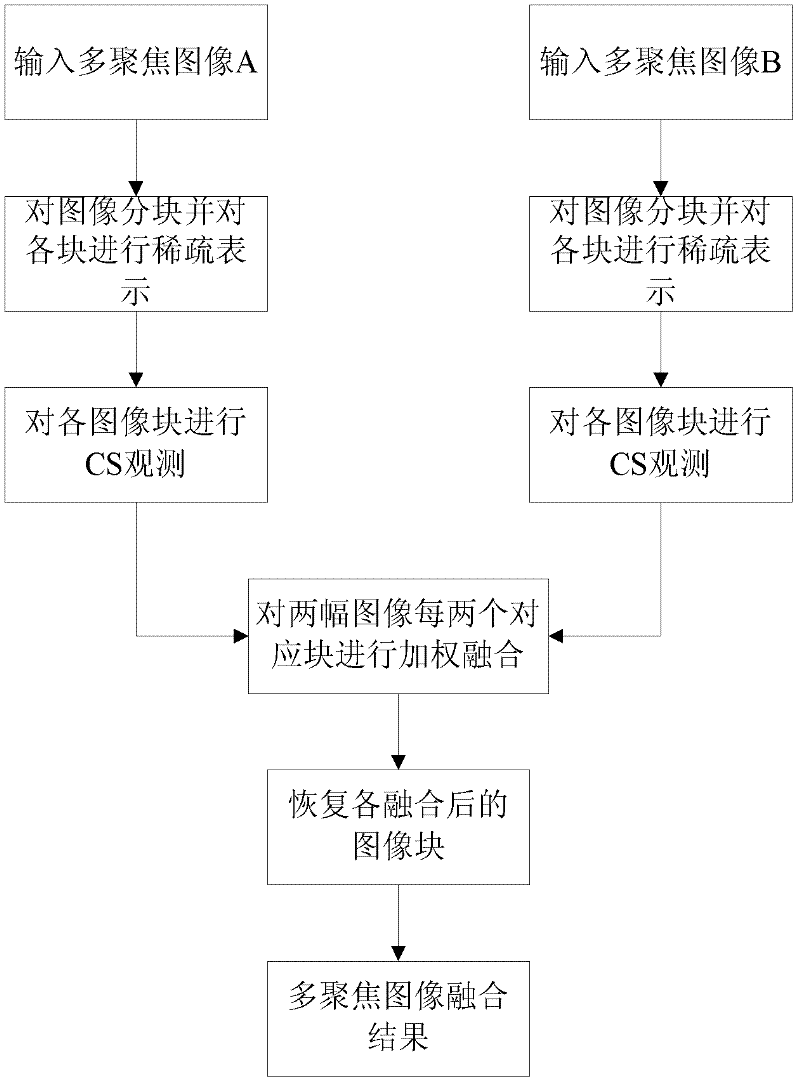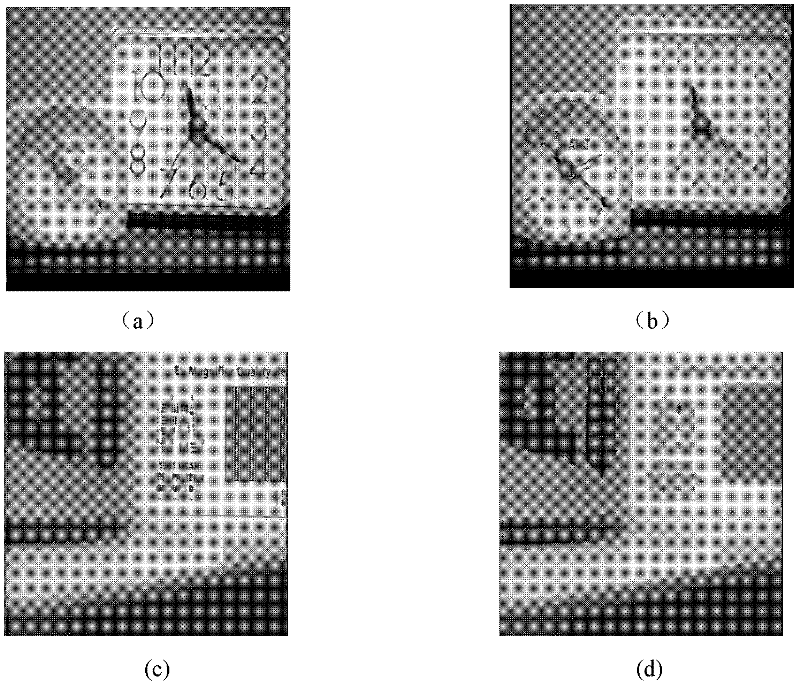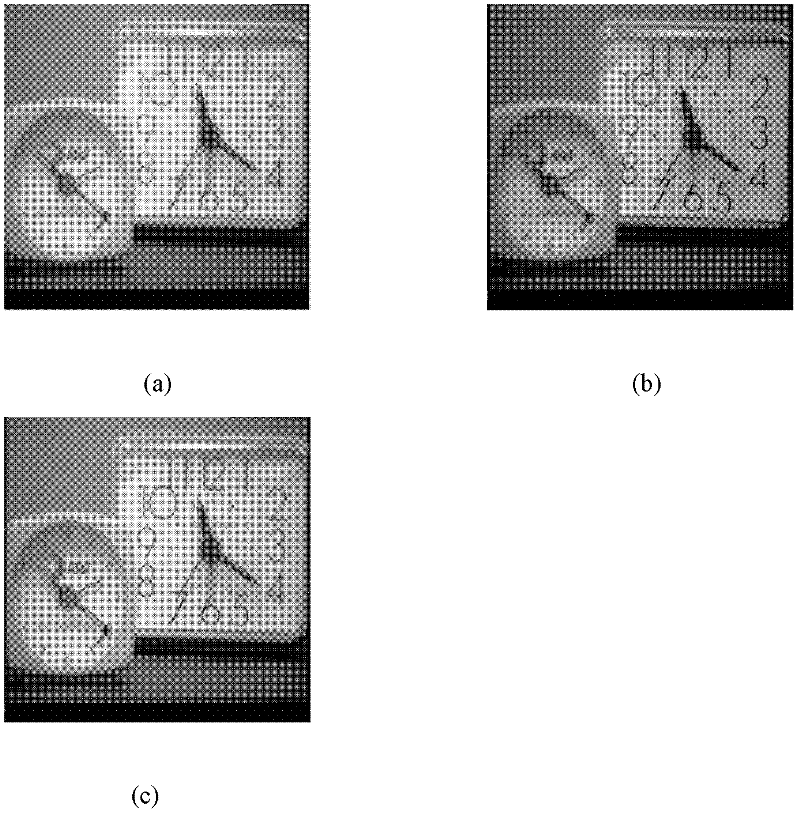# Multi-focus image fusion method based on compressive sensing

## A multi-focus image, compressed sensing technology, applied in the field of image processing, can solve the problems of high computational complexity, noise and stripes in fusion results, and achieve the effect of good visual effect, small amount of data, and saving storage space.

Active Publication Date: 2012-03-28
XIDIAN UNIV
3 Cites 37 Cited by

## AI-Extracted Technical Summary

### Problems solved by technology

However, there are few studies on applying compressive sensing theory to image fusion.
Scholar T.Wan and others took the lead in applying the theory of compressed sensing to image fusion, see the article "Compressive Image Fusi...
the structure of the environmentally friendly knitted fabric provided by the present invention; figure 2 Flow chart of the yarn wrapping machine for environmentally friendly knitted fabrics and storage devices; image 3 Is the parameter map of the yarn covering machine
View more

### Method used

 Images A and B are two left-focused and right-focused images respectively, and the information of the clear parts of the two images is complementary, and the purpose is to obtain a clear all-focus image by fusion. Dividing the image into blocks is beneficial t...
the structure of the environmentally friendly knitted fabric provided by the present invention; figure 2 Flow chart of the yarn wrapping machine for environmentally friendly knitted fabrics and storage devices; image 3 Is the parameter map of the yarn covering machine
View more

## Abstract

The invention discloses a multi-focus image fusion method based on compressive sensing and relates to the technical field of image processing. By the multi-focus image fusion method, the main problem that a clear image with all focused sceneries is difficult to acquire due to a limited depth of field of an optical lens in the prior art can be solved. The multi-focus image fusion method is implemented by the following steps of: (1) blocking an image; (2) calculating an average gradient of each image sub block to determine a fusion weight value; (3) performing sparse representation on each image sub block and observing each image sub block by adopting a random Gaussian matrix; (4) performing weighted fusion on the fusion weight value of an observed value of each image sub block; and (5) recovering a fused image observed value by adopting an orthogonal matching traceback algorithm and performing wavelet inverse transformation on a recovered result to acquire a fused fully-focused image. By the multi-focus image fusion method based on the compressive sensing, a better image fusion effect can be achieved and higher convergence property is realized; and the method can be applied to fusion of a multi-focus image.

Application Domain

Technology Topic

## Image

•••## Examples

• Experimental program(1)

### Example Embodiment

 Reference figure 1 The specific implementation steps of the present invention are as follows:
 Step 1. Divide the input two multi-focus images A and B into blocks and calculate the average gradient of each image sub-block with
 Images A and B are two left-focused and right-focused images, respectively. The clear parts of the two images have complementary information, and the purpose is to obtain an all-focused image with clear left and right sides through fusion. The image is divided into blocks, which is beneficial to processing and can reduce the computational complexity. The present invention divides the two multi-focus images A and B into image sub-blocks with a size of 32×32, and calculates the average gradient of each image sub-block , Calculated according to the following formula:
 ▿ G ‾ I = 1 M X N X i = 1 M X j = 1 N [ Δxf ( i , j ) 2 + Δyf ( i , j ) 2 ] 1 2
 Among them, Δxf (x, y), Δyf (x, y) are the first-order difference of the multi-focus image I sub-block pixel (i, j) in the x, y direction, I=A, B, M×N is Image sub-block size, Is the average gradient of the I sub-block of the multi-focus image.
 Step 2 For each corresponding image sub-block x of multi-focus image A and B i And y i Perform wavelet transformation to get the image sub-block a after sparse transformation i And b i.
 The sparse transformation of each image sub-block of the multi-focus image A and B is to make the signal meet the prerequisite of compressed sensing, that is, as long as the signal is compressible or sparse in a certain transform domain, you can use one and transform The uncorrelated observation matrix projects the transformed high-dimensional signal onto a low-dimensional space, and then by solving an optimization problem, the original signal can be reconstructed with high probability from these few projections. The sparse transform used in this example is a biorthogonal filter CDF 9/7 wavelet transform, and the number of decomposition layers is 3, but it is not limited to wavelet transform. For example, discrete cosine DCT transform, Fourier FT transform, etc. can be used.
 Step 3: Use a random Gaussian matrix to perform CS observation on the corresponding image sub-blocks of the multi-focus images A and B.
 The CS observation of the image is a linear process. In order to ensure accurate reconstruction, the necessary and sufficient condition for the existence of a definite solution of the linear equations is that the observation matrix and the sparse transformation basis matrix satisfy the finite isometric property RIP. The random Gaussian matrix is ​​not related to the matrix composed of most fixed orthogonal bases. This feature determines that it is selected as the observation matrix. When other orthogonal bases are used as the sparse transform base, the RIP property can be satisfied. Therefore, the present invention adopts the random Gaussian matrix as Observation matrix, CS observation of each corresponding image sub-block, the specific operation is as follows:
 (3a) The N×N image sub-blocks corresponding to each of the two multi-focus images A and B are a i And b i Arrange to N 2 ×1 column vector θ A And θ B;
 (3b) Randomly generate M×N 2 Random Gaussian matrix and orthogonalize it, use random Gaussian matrix to observe the column vector, the specific calculation formula is as follows;
 y I =Φθ I
 Among them, Φ is the random Gaussian observation matrix, θ I Is the column vector of the image sub-block, I=A, B, y I Is the observation value of each image sub-block. The sampling rate of each image sub-block in this example is The sampling rate is controlled by adjusting the value of the random Gaussian matrix M.
 Observe each image sub-block of the multi-focus image A and B to obtain the observation value of each image sub-block. The size of the observation value vector is M×1. In the experiment, the same observation is used to observe each image sub-block Matrix Φ.
 Step 4. Perform a weighting method for each two corresponding image sub-blocks of the two multi-focus images A and B.
 The observation values ​​of each image sub-block of the multi-focus image A and B after the random Gaussian matrix observation still retain all the information of the original image sub-block, so the average gradient of the original image sub-block is calculated to determine each image sub-block after observation The fusion weight of the observation value, the average gradient is an evaluation index of image fusion, which reflects the clarity of the image. A clear image block has a large average gradient, and the weight should also be large during fusion.
 The two corresponding image sub-blocks of images A and B are fused by a weighted method, and the specific implementation is as follows:
 (4a) Calculate the fusion weights of each two corresponding image sub-blocks of multi-focus images A and B:
 w A = 0.5 if ▿ G ‾ A = ▿ G ‾ B = 0 ▿ G ‾ A ▿ G ‾ A + ▿ G ‾ B else
 w B = 1-w A
 among them, Are the average gradients of the corresponding image sub-blocks of multi-focus images A and B, w A , W B Are the fusion weights of image sub-blocks corresponding to images A and B;
 (4b) Perform weighted fusion on the observation values ​​of each two corresponding image sub-blocks of the multi-focus images A and B:
 y=w A y A +w B y B
 Where y A , Y B These are the observed values ​​of the two corresponding image sub-blocks of the multi-focus images A and B, and y is the observed value after fusion.
 Step 5: Use the orthogonal matching pursuit algorithm to restore the observed values ​​of the fused image sub-blocks to obtain the restored image sub-blocks.
 Orthogonal matching OMP tracking algorithm is based on greedy iterative algorithm. It uses more samples than the base tracking BP algorithm to reduce the computational complexity. The orthogonal matching tracking OMP algorithm is used to solve the optimization problem and reconstruct the signal, which greatly improves the calculation Speed, and easy to implement. The specific operation is to restore the fused image blocks one by one. For the specific steps of the algorithm, see "Signal Recovery From Random Measurements Via Orthogonal Matching Pursuit", IEEE Transactions on Information Theory, vol.53, No .12, December 2007.
 Step 6. Perform wavelet inverse transformation on the image sub-blocks of the multi-focus images A and B restored by the orthogonal matching pursuit algorithm.
 The data restored by the orthogonal matching pursuit algorithm is the sparse form of the fused all-focus image. The inverse wavelet transform is performed on each restored image sub-block to obtain the fused all-focus image sub-block. Combining the blocks into one image can get the fused all-in-focus image.
 The effects of the present invention can be specifically illustrated through simulation experiments:
 1. Experimental conditions
 The microcomputer CPU used in the experiment is Intel Core(TM) 2Duo 2.33GHz memory 2GB, and the programming platform is Matlab7.0.1. The image data used in the experiment are two sets of registered multi-focus images with sizes of 512×512 and 512×512 respectively. The two sets of multi-focus source images come from the image fusion website http:∥www.imagefusion.org/ , The first group is Clock images, such as figure 2 (a) and figure 2 (b), where figure 2 (a) is the source image with Clock focused on the right, figure 2 (b) is the source image with Clock focused on the left, the second group is the Pepsi image, such as figure 2 (c) and figure 2 (d), where figure 2 (c) is the source image with Pepsi focused on the right, figure 2 (d) is the source image with Clock focused on the left.
 2. Experimental content
 (2a) Use the method of the present invention and the two existing fusion methods to perform fusion experiments on Clock images, and the sampling rate of each group is set to 0.3, 0.5, 0.7, and the fusion results are as follows image 3 ,among them image 3 (a) is the fusion result diagram of the existing average method, image 3 (b) is the fusion result image of the article "Compressive Image Fusion", in Proc.IEEE Int.Conf.Image Process, pp.1308-1311, 2008, image 3 (c) is the fusion result graph of the present invention, and the sampling rate of the three sets of graphs is 0.5.
 (2b) Use the method of the present invention and the two existing fusion methods to perform fusion experiments on Pepsi images, and the sampling rate of each group is set to 0.3, 0.5, 0.7, and the fusion results are as follows Figure 4 ,among them Figure 4 (a) is the fusion result diagram of the existing average method, Figure 4 (b) is the fusion result image of the article "Compressive Image Fusion", in Proc.IEEE Int.Conf.Image Process, pp.1308-1311, 2008, Figure 4 (c) is the fusion result graph of the present invention, and the sampling rate of the three groups of graphs is 0.5. The averaging method has the same operation as the method of the present invention, but the fusion rules are different. The fusion weight of the averaging method is w A =w B = 0.5.
 3. Experimental results
 Compare the fusion method of the present invention with the weighted average method and the method of the article "Compressive Image Fusion", in Proc. IEEE Int. Conf. Image Process, pp. 1308-1311, 2008. on three image evaluation indicators, to Evaluate the effect of the present invention. The fusion method and weighted average method of the present invention and the article "Compressive Image Fusion", in Proc. IEEE Int. Conf. Image Process, pp. 1308-1311, 2008. Qualitative evaluation index for the fusion of two sets of multi-focus images As shown in Table 1:
 Table 1. Qualitative evaluation indicators for multi-focus image fusion

 In Table 1, Mean CS-max-abs Ours are the existing average method, the article "Compressive Image Fusion", in Proc.IEEE Int.Conf.Image Process, pp.1308-1311, 2008. The method and the method of the present invention. ; R is the sampling rate, MI is the mutual information, IE is the information entropy, Q is the edge retention, and T is the time required for image reconstruction, in seconds (s). among them:
 Mutual information (MI): Mutual information reflects how much information the fused image extracts from the original image. The greater the amount of mutual information, the more information can be extracted.
 Information Entropy (IE): Image information entropy is an important indicator to measure the richness of image information. The size of entropy reflects the amount of information carried by the image. The larger the entropy value, the greater the amount of information carried.
 Edge retention (Q): It is essentially a measure of the degree of retention of the edge information in the input image by the fused image. The value range is 0 to 1. The closer to 1, the better the degree of edge retention.
 From the data in Table 1, it can be seen that in terms of performance index, the edge retention Q index of the method of the present invention is currently higher than that of the average method and the CS-max-abs method. In terms of the mutual information MI index, the method of the present invention is better than the average method. Higher, and much higher than the CS-max-abs method. The information entropy IE index is equivalent to the average method, but lower than the CS-max-abs method. In terms of image reconstruction time T, the method of the present invention requires much less time than the CS-max-abs fusion method. As the sampling rate increases, the indicators of the fusion result gradually increase.
 From Figure 3-Figure 4 It can be seen that the fusion result of the fusion method of the present invention on the two sets of multi-focus images is better than the average method and the CS-max-abs fusion method. The fusion result of the CS-max-abs fusion method has a lot of noise and striped stripes. , The contrast is also low. The visual effect of the CS-max-abs fusion method is not as good as the method of the present invention, but the information entropy IE index is higher than the method of the present invention because noise is generated during the fusion process, which causes the information entropy IE index to not truly reflect the useful information of the fused image the amount.
 The above experiments prove that the multi-focus image fusion method based on compressed sensing proposed by the present invention can achieve good visual effects for the multi-focus image fusion problem, and the computational complexity is also low.
the structure of the environmentally friendly knitted fabric provided by the present invention; figure 2 Flow chart of the yarn wrapping machine for environmentally friendly knitted fabrics and storage devices; image 3 Is the parameter map of the yarn covering machine

## PUM## Description & Claims & Application Information

We can also present the details of the Description, Claims and Application information to help users get a comprehensive understanding of the technical details of the patent, such as background art, summary of invention, brief description of drawings, description of embodiments, and other original content. On the other hand, users can also determine the specific scope of protection of the technology through the list of claims; as well as understand the changes in the life cycle of the technology with the presentation of the patent timeline. Login to view more.
the structure of the environmentally friendly knitted fabric provided by the present invention; figure 2 Flow chart of the yarn wrapping machine for environmentally friendly knitted fabrics and storage devices; image 3 Is the parameter map of the yarn covering machine

Owner:小米汽车科技有限公司

## Method and device for aligning machine vision

Owner:SHANGHAI UNIV

## Method for publishing fault repair data packet and server

ActiveCN108322345AReduce data volumeReduced hardware and bandwidth requirementsData switching networksNetwork packetComputer science
Owner:PING AN TECH (SHENZHEN) CO LTD

## Method of data transmission with differential data fusion

Owner:INSTITUTE FOR INFORMATION INDUSTRY

## Gesture identification method and electronic equipment

Owner:LENOVO (BEIJING) CO LTD

## Classification and recommendation of technical efficacy words

• Save storage space
• Reduce data volume

Owner:IBM CORP

## Video commenting method and video player

Owner:GLOBAL INNOVATION AGGREGATORS LLC

## System and method for copying and transmitting telephony conversations

Owner:CISCO TECH INC

## System and method for restaurant electronic menu

InactiveUS20060085265A1Save storage spaceFast retrievalMarketingSoftwareNutritional information
Owner:IBM CORP

## System recovery method and embedded system with automatic recovery function

Owner:VIVOTEK INC
Who we serve
• R&D Engineer
• R&D Manager
• IP Professional
Why Eureka